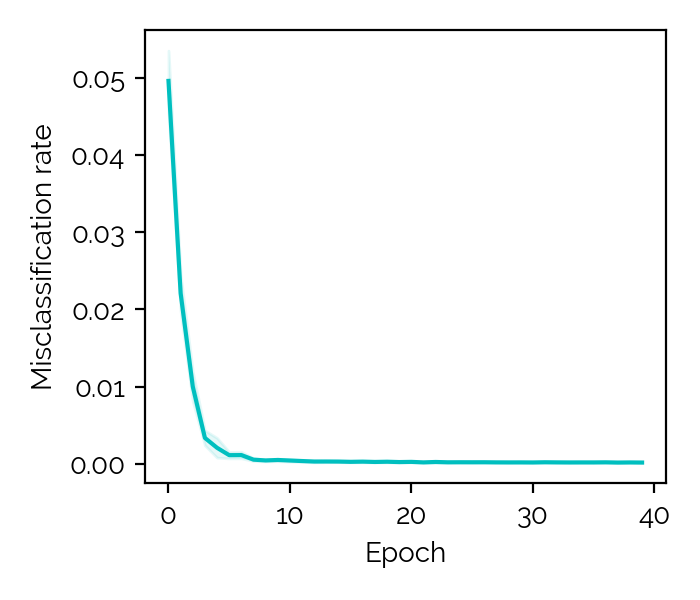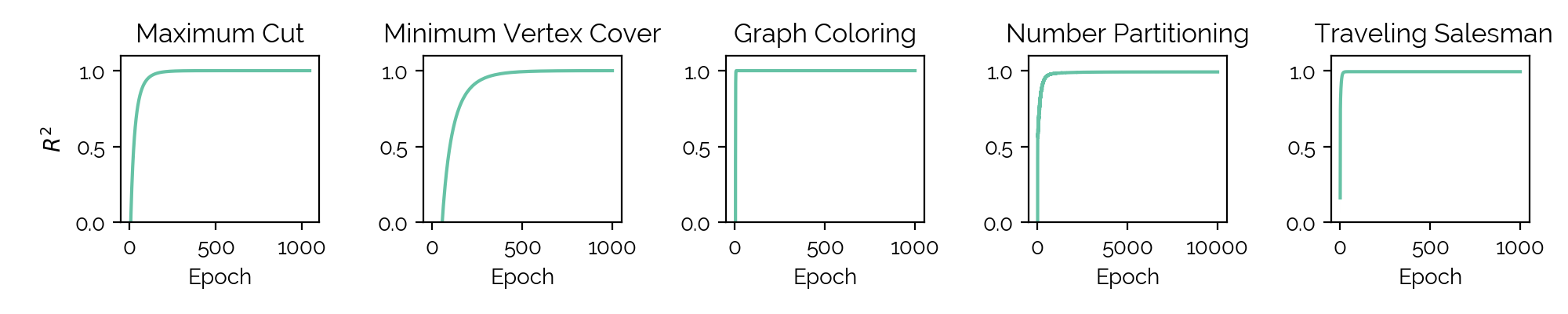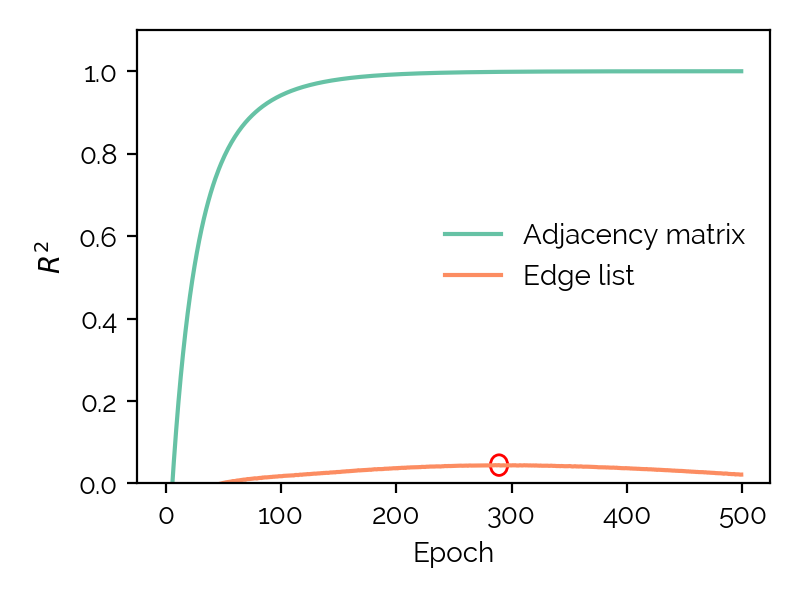## # QUBO NN - Reverse Engineering QUBO matrices

I've been trying to create a reverse-engineering pipeline for QUBO matrices, and it has been quite interesting.. Not as easy as one would think. So the story here is quite simple: Since the current global Quantum Compute is concentrated across a few companies and institutions, what if these institutions read your QUBO matrices and infer secret information. For instance, say you're a hedge fund that quantifies some secret alpha - they trust you not to steal that and thereby instantly devalue their alpha.

In classical compute (with cloud computing) there are interesting ideas such as homomorphic computation (using homomorphic encryption). The goal of the project is to create a pipeline that can classify the problem a given QUBO matrices is based on and then infer the parameters that the QUBO matrix has been generated with. Below a schematic overview is given.I used neural networks for both steps. We do neural network multi-classification (step a) and neural network regression (step b). Now, the results are quite promising.

The first step, i.e. classifying, is pretty straightforward. I set up 11 problems and generated 100000 problem instances each. The chart below shows how after just 40 epochs we are already near 0 in terms of error rate:The second step is not as straightforward. 5 of the problems turned out to be trivially reversible. The $R^2$ coefficient comes very close to 1.0:Maximum Cut needs additional thoughts (the blog post about that can be found here) - specifically, the output needs to be an adjacency matrix and not an edge list. This is actually very important:As for the rest, I am actively looking into it. A table with all 11 problems and their reversibility can be found here. Some problems such as Quadratic Assignment (QA) I assumed to be non-reversible, but I was wrong. The QUBO of a QA problem defines an over-determined linear system of equations, which can be solved by a neural network. However, the best I got so far is a $R^2$ coefficient of 99%. Not bad, but could be better. The problem I really struggled with so far is Maximum 2-SAT. I am not 100% sure yet, but my hunch is that it is simply too complex for a standard fully connected neural network.

For anyone interested, the Github repository can be found here.

An update post can be found here.

Published on